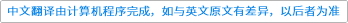International Journal of Mathematics ( IF 0.604 ) Pub Date : 2020-07-31 , DOI: 10.1142/s0129167x20500809
M. Bischoff

Using a result of Longo and Xu, we show that the anomaly arising from a cyclic permutation orbifold of order 3 of a holomorphic conformal net $𝒜$ with central charge $c=8k$ depends on the “gravitational anomaly” $k(mod3)$. In particular, the conjecture that holomorphic permutation orbifolds are non-anomalous and therefore a stronger conjecture of Müger about braided crossed $Sn$-categories arising from permutation orbifolds of completely rational conformal nets are wrong. More generally, we show that cyclic permutations of order $n$ are non-anomalous if and only if $3∤n$ or $24|c$. We also show that all cyclic permutation gaugings of $Rep(𝒜)$ arise from conformal nets.down
wechat
bug